# 前言

1.24号考了这个学期的第三次GRE考试，虽然结果不是特别理想超出预料,但是也不错了．

155+167

# 奥数题目

##1.看题库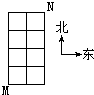## 2.看题库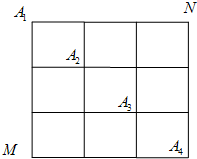（2）由（1）知，甲经过A2的方法数为 $(C_3^1)^2$ ；

$P=\frac{(C_3^1)^4}{C_6^3 C_6^3}=\frac{81}{400}$

（3）甲、乙两人沿最短路径行走，只可能在A1、A2、A3、A4处相遇，

$(C_3^0)^4 +(C_3^1)^4 +(C_3^2)^4 +(C_3^3)^4 =164$

（2）甲、乙两人在A2处相遇的概率为 $\frac{81}{400}$

（3）甲、乙两人相遇的概率 $\frac{41}{100}$．

# 怎么做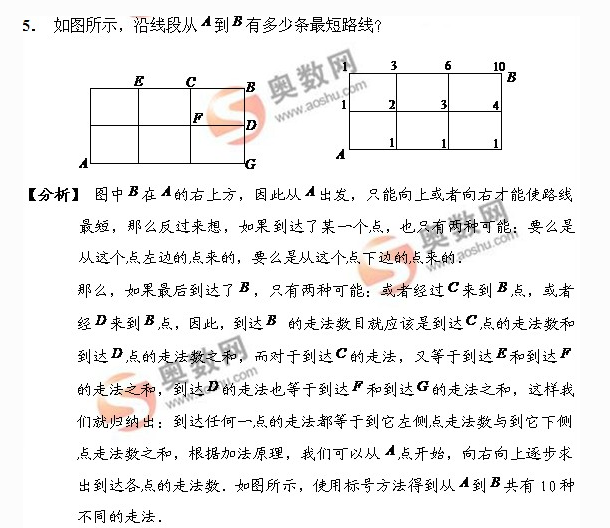# 升华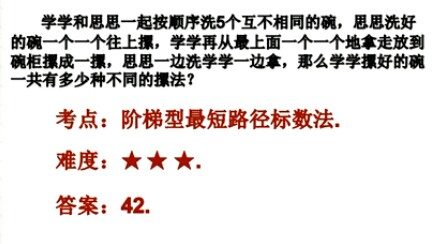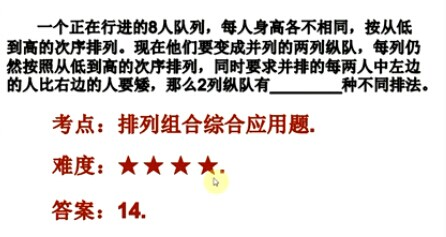Written on January 26, 2015